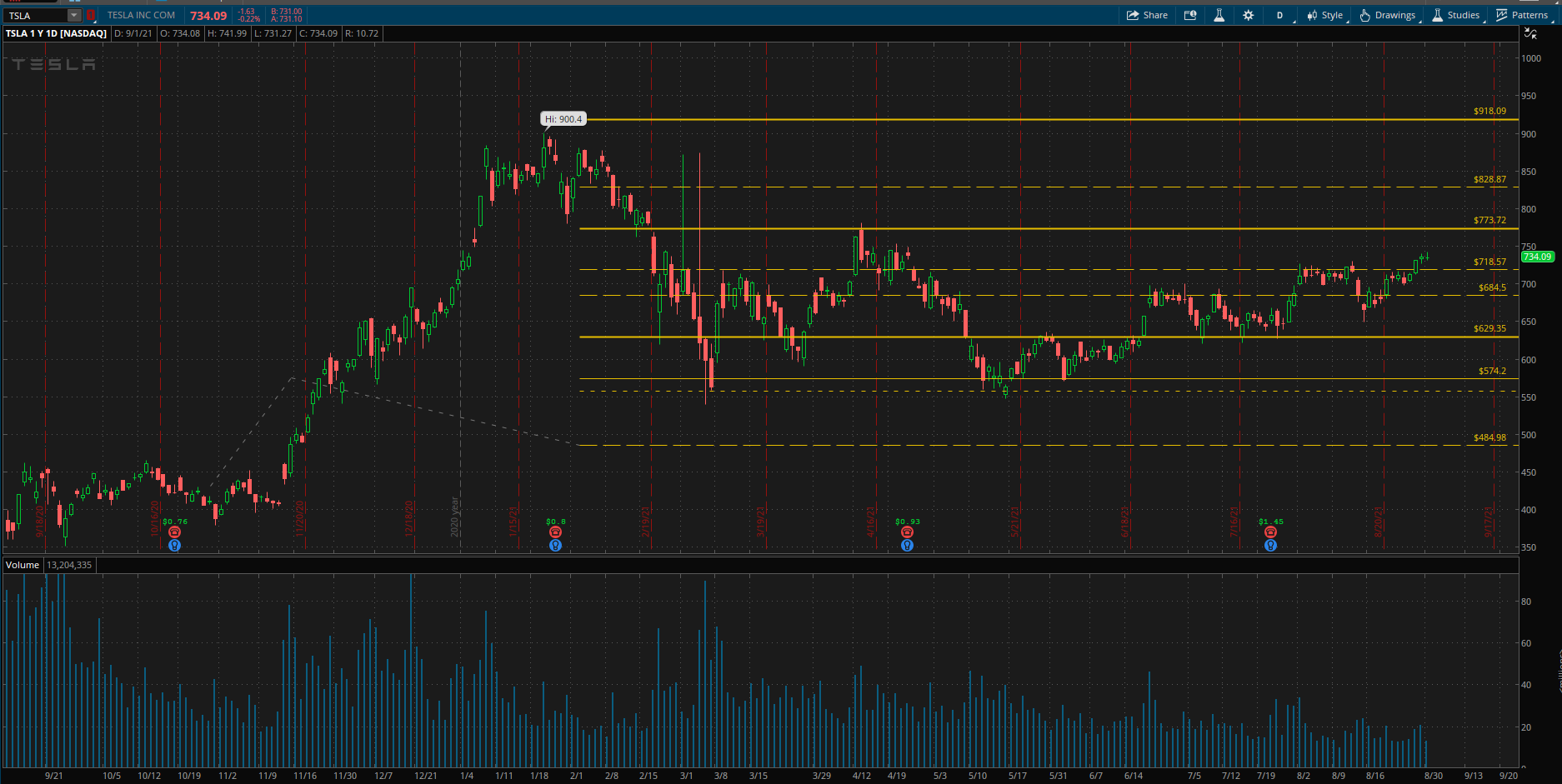# What Are Fibonacci Extensions?

Fibonacci extensions are a tool that traders can use to establish potential price targets or estimate how far the stock price may move. Extension levels are also possible areas where the price may reverse.

• Common Fibonacci extension levels are 61.8%, 100%, 161.8%, 200%, and 261.8%
• The Fibonacci extensions show how far the next price wave could move following a pullback.
• Fibonacci ratios are common in everyday life, seen in galaxy formations, architecture, and how some plants grow. Therefore, some traders believe these common ratios may also have significance in the financial markets.
• Extension levels signal possible areas of importance but should not be relied on exclusively.

## The Formula for Fibonacci Extensions

There are three points that a trader has to choose from.

1. The first point chosen is the start of a move
2. The second point is the end of a move
3. The third point is the end of the retracement and the base of where the calculations will start.

The extensions then help project where the price could go next. Once the three points are chosen, the lines are drawn at percentages of that move.

Extensions are drawn on a chart, marking price levels of possible importance.## How to Calculate Fibonacci Levels

1. Find the difference between your first point and your second point. This will be the “range” of your Fibonacci.
2. Multiply the difference between points one and two by any of the ratios desired, such as 1.618 or 0.618. This gives you a dollar amount.
3. If projecting a price move higher, add the dollar amount above to the price at point three. If projecting a price move lower, subtract the dollar amount from step one from the price at point three.

## For example, using the Tesla chart provided above:

1. Point one is \$430.91; point two is \$575.28. This makes the range \$144.37.
2. Our point three is \$485. If we wanted to find what the 61.8% or the 0.618 retracement level is, you would do the following:
1. Multiply the range, \$144.37, by the coefficient of 0.618
2. This will give you \$89.22
3. To find the level 61.8% level above \$485, you will add \$89.22, and if you want the 61.8% level below \$485, you will subtract \$89.22
4. The results are: \$574.22 and \$395.78
1. Do this for your desired ratios. I personally enjoy using the 0.5, 0.618, 1.382, 1.618 and 2.382

## What Do Fibonacci Extensions Tell You?

Fibonacci extensions are a way to establish price targets or find projected areas of support or resistance when the price moves into an area where other methods of finding support or resistance are not applicable or evident. If the price moves through one extension level, it may continue moving toward the next. That said, Fibonacci extensions are areas of possible resistance and support. The price may not stop or reverse right at the level, but the area around it may be important. For example, the price may move just past the 1.618 level or pull up just shy of it before changing directions. Fibonacci extensions can be used for any timeframe or in any market. Typically, clusters of Fibonacci levels indicate a price area that will be significant for the stock and traders in their decision-making. Since extension levels can be drawn on different price waves over time, when multiple levels from these different waves converge at one price, that could be a critical area.

## The Difference Between Fibonacci Extensions and Fibonacci Retracements

While extensions show where the price will go following a retracement, Fibonacci retracement levels indicate how deep a retracement could be. In other words, Fibonacci retracements measure the pullbacks within a trend, while Fibonacci extensions measure the impulse waves in the direction of the trend. Limitations of Using Fibonacci Extensions Fibonacci extensions are not meant to be the sole determinant of whether to buy or sell a stock. Investors should use extensions and other indicators or patterns when determining one or multiple price targets. Candlestick patterns and price action are especially informative when determining whether a stock is likely to reverse at the target price. There is no assurance price will reach or reverse at a given extension level. Even if it does, it is not evident before a trade is taken which Fibonacci extension level will be important. The price could move through many of the levels with ease or not reach any of them.